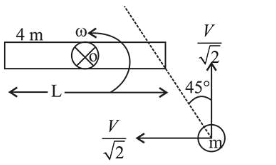# Consider a uniform rod of massQuestion:

Consider $a$ uniform rod of mass $M=4 \mathrm{~m}$ and length $l$ pivoted about its centre. $A$ mass $m$ moving with velocity

$v$ making angle $\theta=\frac{\pi}{4}$ to the rod's long axis collides with one end of the rod and sticks to it. The angular speed of the rod-mass system just after the collision is:

1. (1) $\frac{3}{7 \sqrt{2}} \frac{v}{l}$

2. (2) $\frac{3}{7} \frac{v}{l}$

3. (3) $\frac{3 \sqrt{2}}{7} \frac{v}{l}$

4. (4) $\frac{4}{7} \frac{v}{l}$

Correct Option: 3,

Solution:About point $\mathrm{O}$ angular momentum

$\mathrm{L}_{\text {initial }}=\mathrm{L}_{\text {final }}$

$\Rightarrow \frac{m V}{\sqrt{2}} \times \frac{1}{2}=\left[\frac{4 m L^{2}}{12}+\frac{m L^{2}}{4}\right] \times \omega$

$\therefore \omega=\frac{6 V}{7 \sqrt{2 L}}=\frac{3 \sqrt{2} V}{7 L}$